# Inheritance Andy Wang Object Oriented Programming in C

• Slides: 50Inheritance Andy Wang Object Oriented Programming in C++ COP 3330Hierarchical Relationships • Many types of classes share similarities • Similar private member data • Similar member functions • Inheritance • Factoring out common features into a single base class • Build derived classes that will share or inherit all of the data and functionality of the base class • Except constructors, destructors, and assignment operators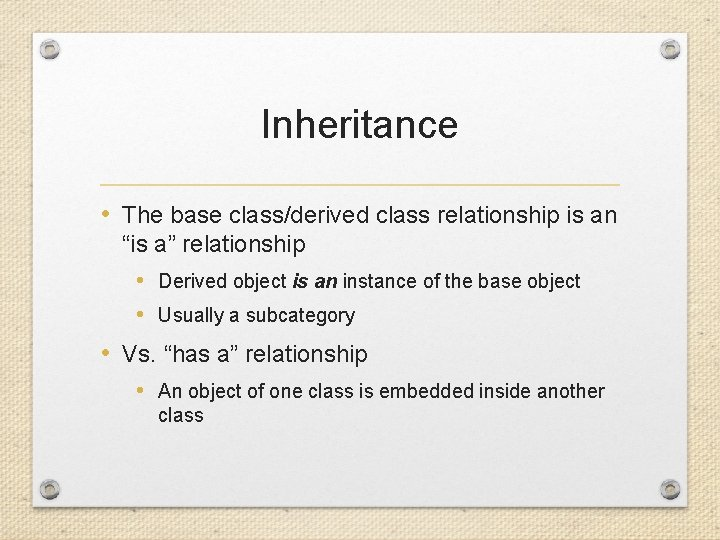Inheritance • The base class/derived class relationship is an “is a” relationship • Derived object is an instance of the base object • Usually a subcategory • Vs. “has a” relationship • An object of one class is embedded inside another classExamples Base class Derived class • • • Geometric. Object Sport Bank. Account Vehicle Car Circle, Square, Line Football, Baseball Savings, Checking Car, Train, Bus Honda, Toyota, Ford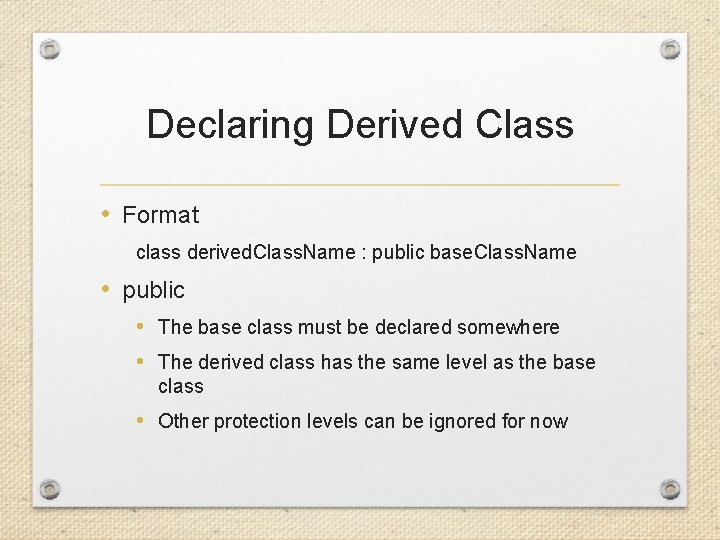Declaring Derived Class • Format class derived. Class. Name : public base. Class. Name • public • The base class must be declared somewhere • The derived class has the same level as the base class • Other protection levels can be ignored for now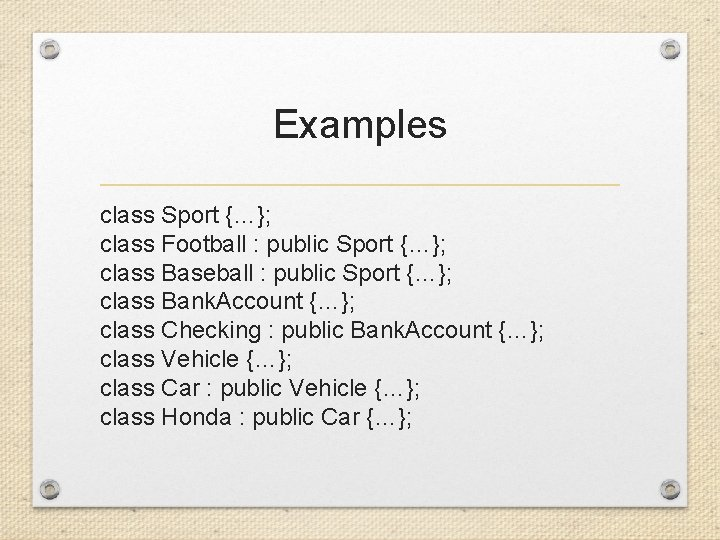Examples class Sport {…}; class Football : public Sport {…}; class Baseball : public Sport {…}; class Bank. Account {…}; class Checking : public Bank. Account {…}; class Vehicle {…}; class Car : public Vehicle {…}; class Honda : public Car {…};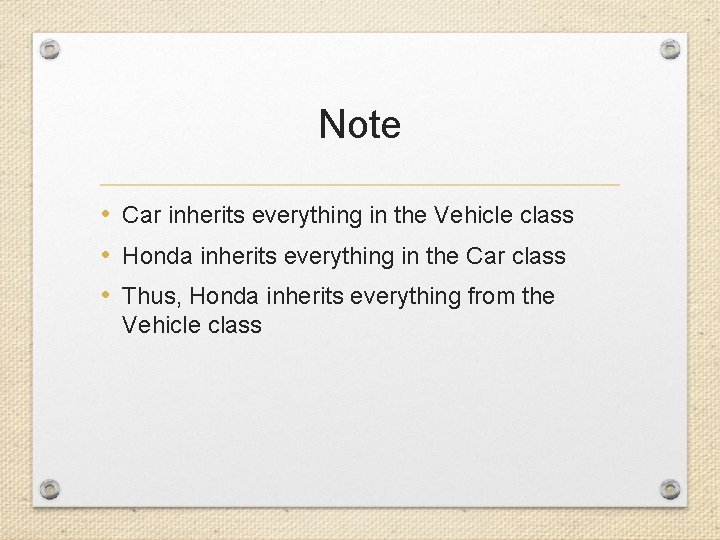Note • Car inherits everything in the Vehicle class • Honda inherits everything in the Car class • Thus, Honda inherits everything from the Vehicle class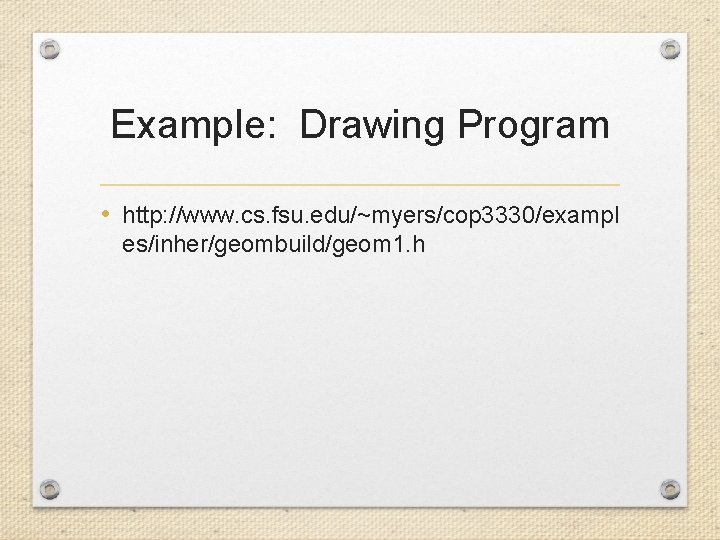Example: Drawing Program • http: //www. cs. fsu. edu/~myers/cop 3330/exampl es/inher/geombuild/geom 1. h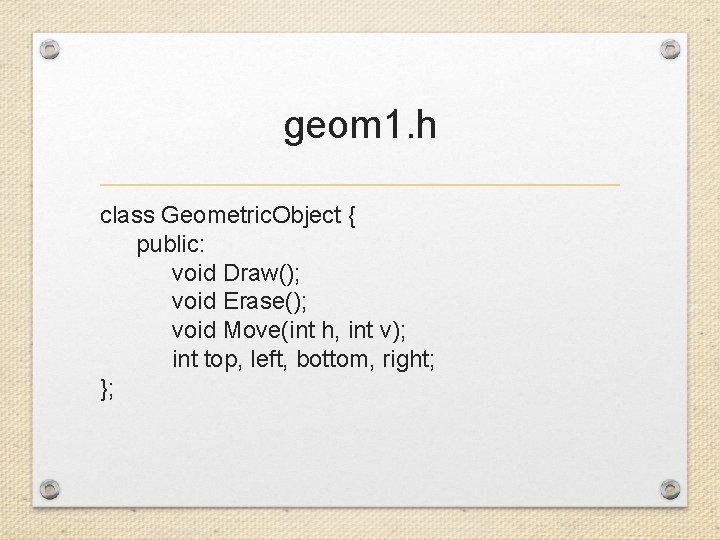geom 1. h class Geometric. Object { public: void Draw(); void Erase(); void Move(int h, int v); int top, left, bottom, right; };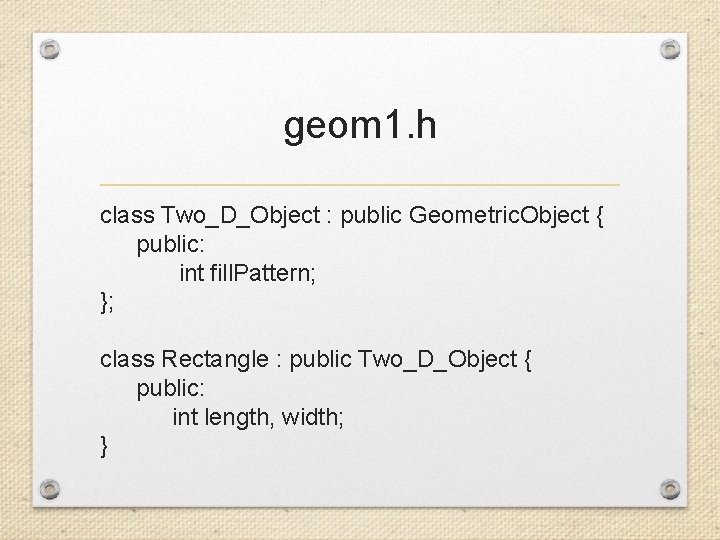geom 1. h class Two_D_Object : public Geometric. Object { public: int fill. Pattern; }; class Rectangle : public Two_D_Object { public: int length, width; }Protection Levels • Public • Can be accessed by name from anywhere • Private • Can be accessed directly only by the class in which it is declared • Protected • Can be accessed by directly by the class in which is declared and by any classes derived from that class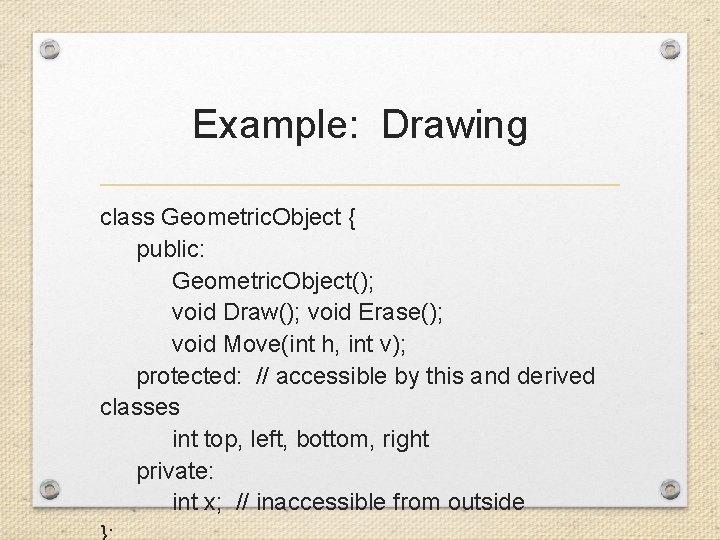Example: Drawing class Geometric. Object { public: Geometric. Object(); void Draw(); void Erase(); void Move(int h, int v); protected: // accessible by this and derived classes int top, left, bottom, right private: int x; // inaccessible from outside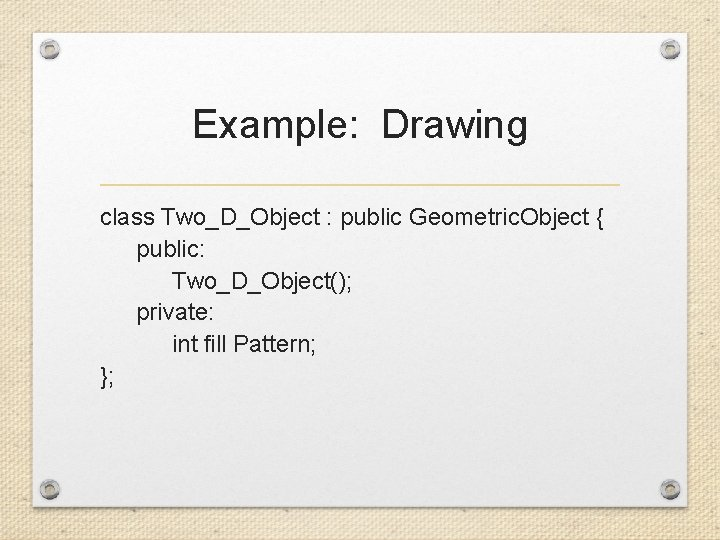Example: Drawing class Two_D_Object : public Geometric. Object { public: Two_D_Object(); private: int fill Pattern; };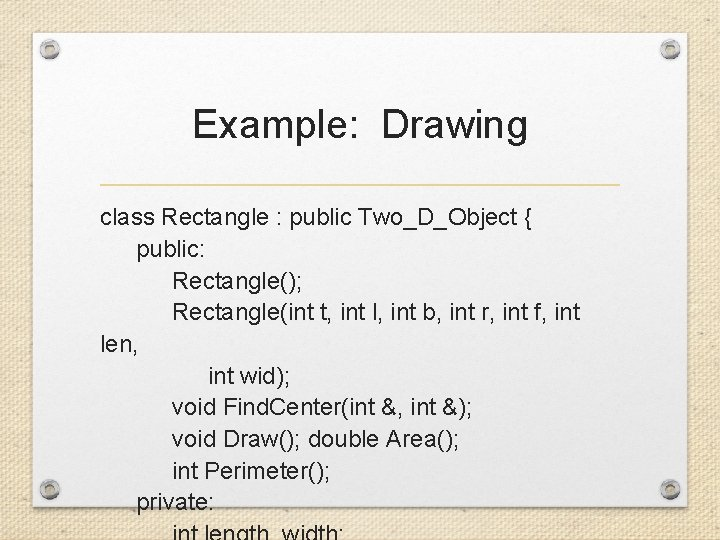Example: Drawing class Rectangle : public Two_D_Object { public: Rectangle(); Rectangle(int t, int l, int b, int r, int f, int len, int wid); void Find. Center(int &, int &); void Draw(); double Area(); int Perimeter(); private:Example: Drawing class Circle : public Two_D_Object { public: Circle(); Circle(int t, int l, int b, int r, int f, int cx, int cy, int rad); void Find. Center(int &, int &); void Draw(); double Area(); double Circumference(); private: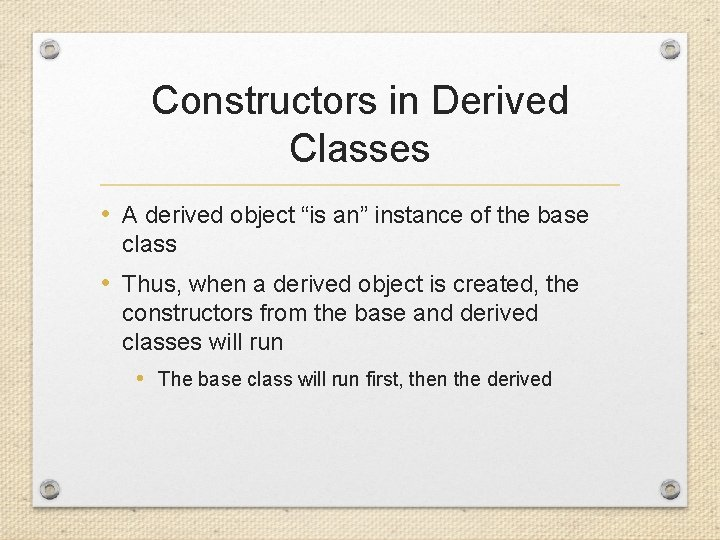Constructors in Derived Classes • A derived object “is an” instance of the base class • Thus, when a derived object is created, the constructors from the base and derived classes will run • The base class will run first, then the derived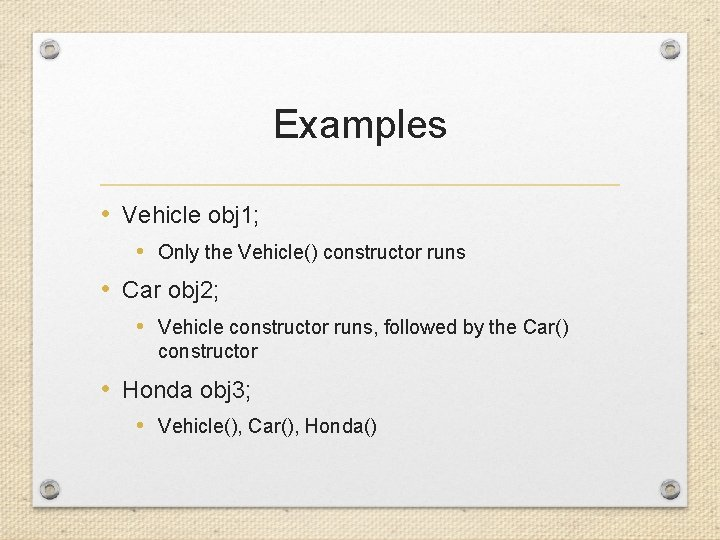Examples • Vehicle obj 1; • Only the Vehicle() constructor runs • Car obj 2; • Vehicle constructor runs, followed by the Car() constructor • Honda obj 3; • Vehicle(), Car(), Honda()Note • Destructors will be invoked in the reverse order • ~Honda(), ~Car(), ~Vehicle()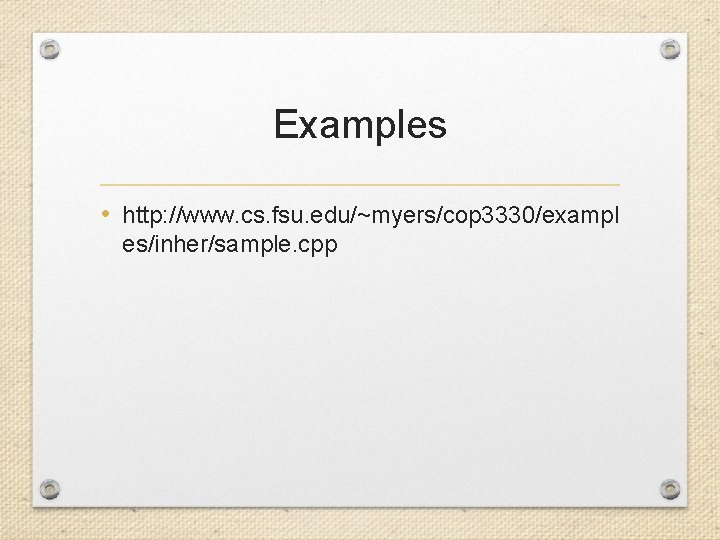Examples • http: //www. cs. fsu. edu/~myers/cop 3330/exampl es/inher/sample. cppInheritance #include <iostream> using namespace std; class A { public: A() { cout << “A()” << endl; } ~A() { cout << “~A” << endl; } };Inheritance class B : public A { public: B() { cout << “B()” << endl; } ~B() { cout << “~B()” << endl; } }; class C : public B { public: C() { cout << “C()” << endl; } ~C() { cout << “~C()” << endl; } };Inheritance int main() { cout << “Declaring object of type A” << endl; A aobject; { cout << “Declaring object of type B” << endl; B bobject; { cout << Declaring object of type C” << endl; C cobject;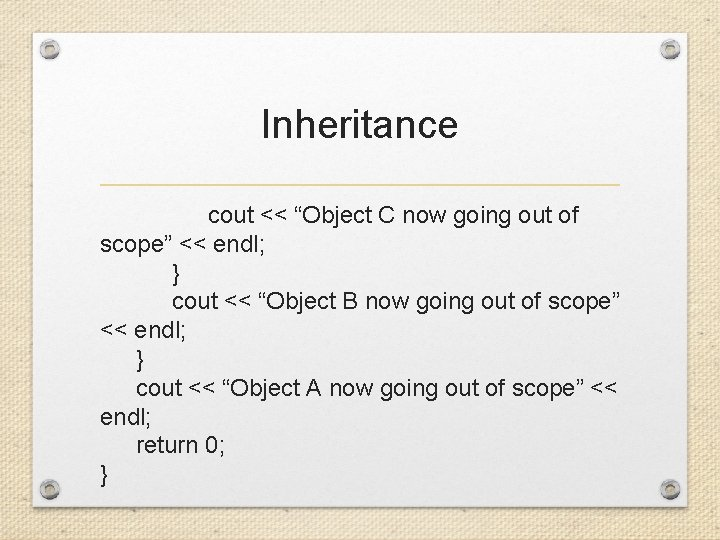Inheritance cout << “Object C now going out of scope” << endl; } cout << “Object B now going out of scope” << endl; } cout << “Object A now going out of scope” << endl; return 0; }Constructors with Parameters • Without parameters, the default constructors are called • With a derived class, we might want to declare • Honda h(2, 3, 4, “green”, “Accord”); • 2 and 3 might be for variables like vehicle. Category and id. Number in the Vehicle Class • 4 and “green” might be for variables like num. Doors and car. Color in the Car Class • “Accord” might be for a variable model in the Honda class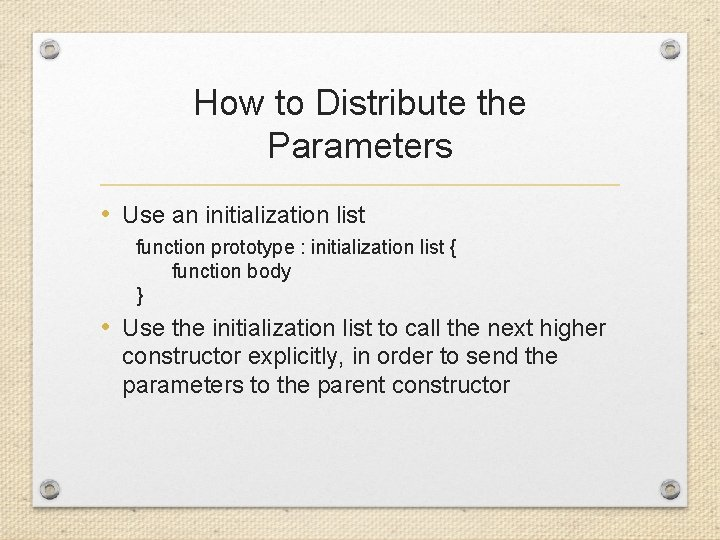How to Distribute the Parameters • Use an initialization list function prototype : initialization list { function body } • Use the initialization list to call the next higher constructor explicitly, in order to send the parameters to the parent constructor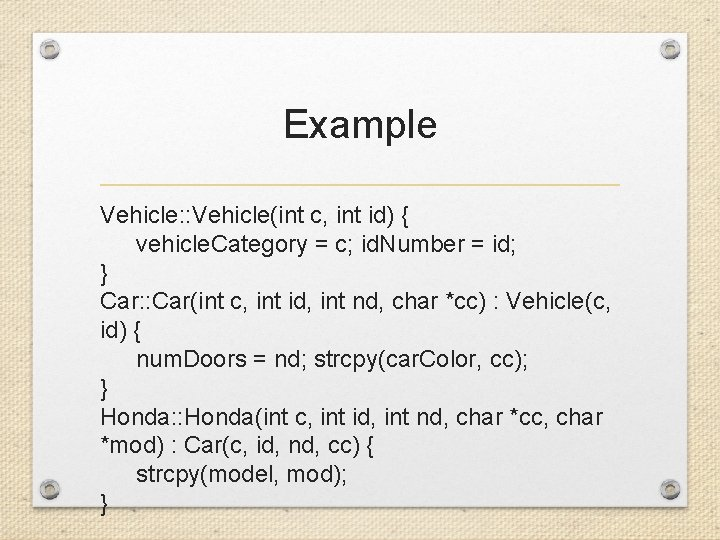Example Vehicle: : Vehicle(int c, int id) { vehicle. Category = c; id. Number = id; } Car: : Car(int c, int id, int nd, char *cc) : Vehicle(c, id) { num. Doors = nd; strcpy(car. Color, cc); } Honda: : Honda(int c, int id, int nd, char *cc, char *mod) : Car(c, id, nd, cc) { strcpy(model, mod); }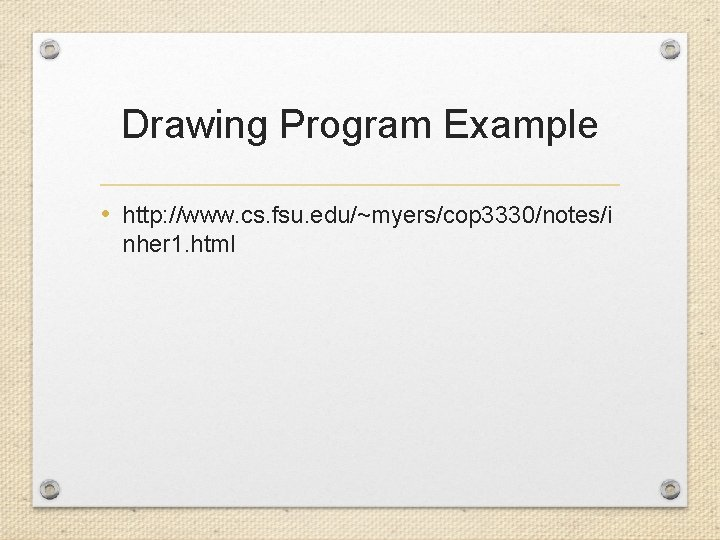Drawing Program Example • http: //www. cs. fsu. edu/~myers/cop 3330/notes/i nher 1. html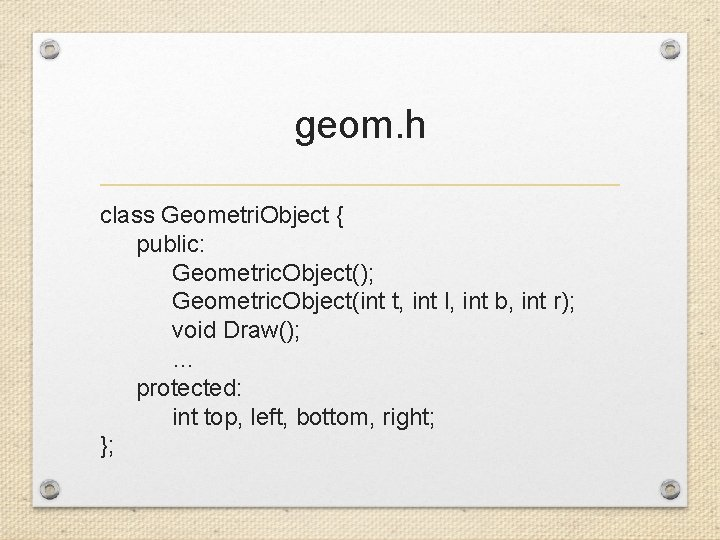geom. h class Geometri. Object { public: Geometric. Object(); Geometric. Object(int t, int l, int b, int r); void Draw(); … protected: int top, left, bottom, right; };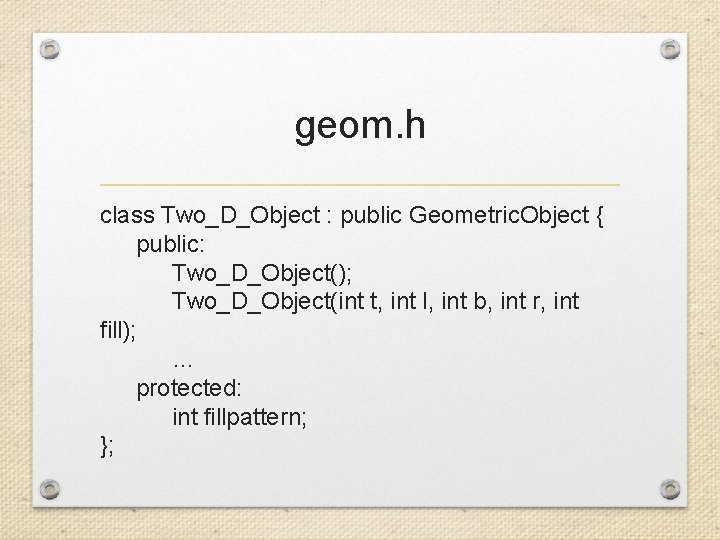geom. h class Two_D_Object : public Geometric. Object { public: Two_D_Object(); Two_D_Object(int t, int l, int b, int r, int fill); … protected: int fillpattern; };geom. h class Rectangle : public Two_D_Object { public: Rectangle(); Rectangle(int t, int l, int b, int r, int f, int len, int wid); … private: int length, width; };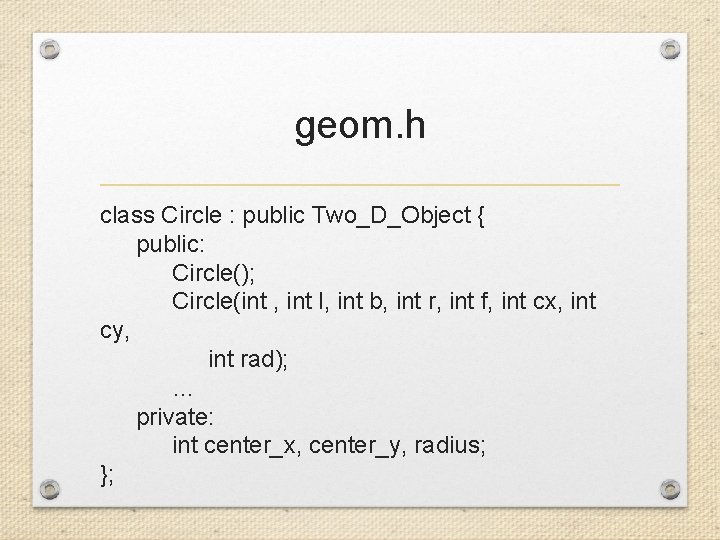geom. h class Circle : public Two_D_Object { public: Circle(); Circle(int , int l, int b, int r, int f, int cx, int cy, int rad); … private: int center_x, center_y, radius; };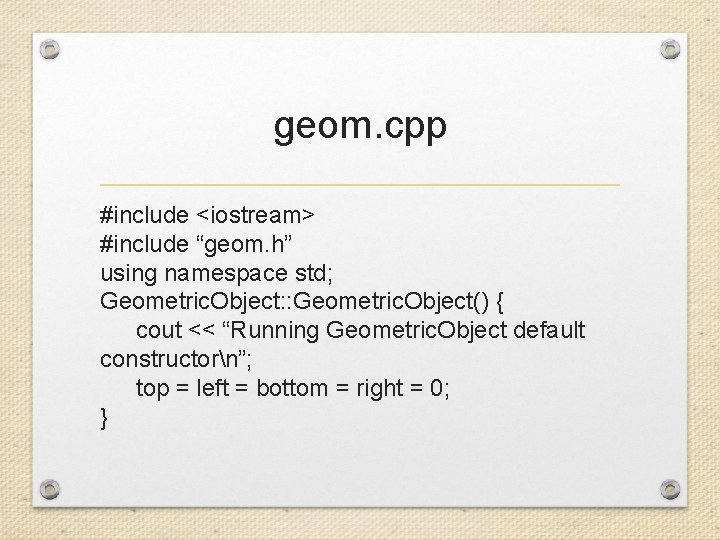geom. cpp #include <iostream> #include “geom. h” using namespace std; Geometric. Object: : Geometric. Object() { cout << “Running Geometric. Object default constructorn”; top = left = bottom = right = 0; }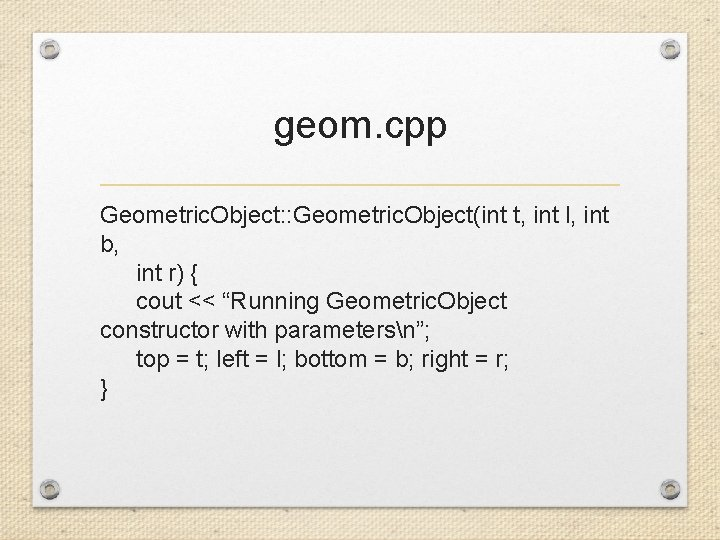geom. cpp Geometric. Object: : Geometric. Object(int t, int l, int b, int r) { cout << “Running Geometric. Object constructor with parametersn”; top = t; left = l; bottom = b; right = r; }geom. cpp Two_D_Object: : Two_D_Object() { cout << “Running Two_D_Object default constructorn”; fill. Pattern = 0; } Two_D_Object: : Two_D_Object(int t, int l, int b, int r, int fill) : Geometric. Object(t, l, b, r) { cout << “Running Two_D_Object constructor with parametersn”; fill. Pattern = fill; }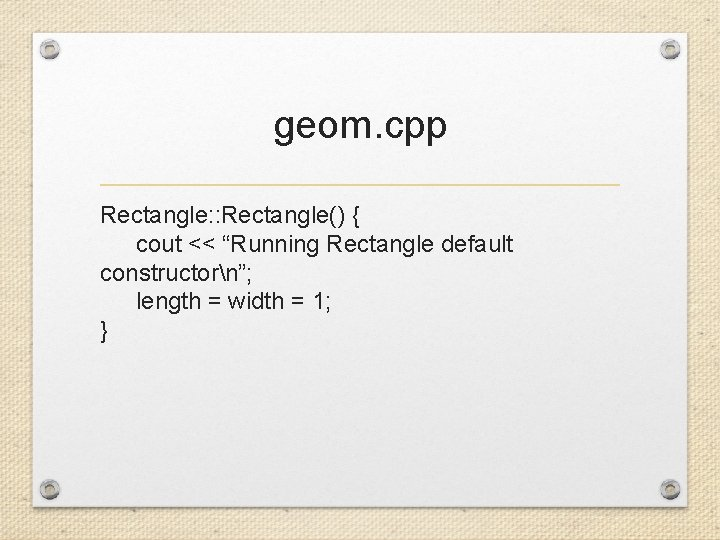geom. cpp Rectangle: : Rectangle() { cout << “Running Rectangle default constructorn”; length = width = 1; }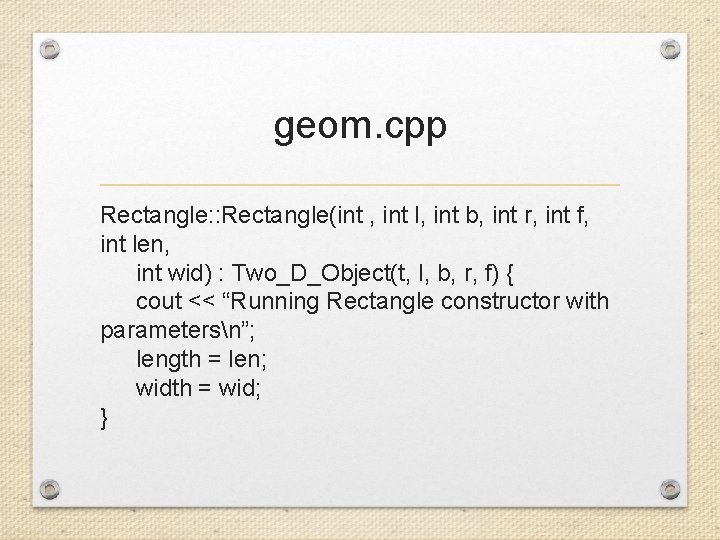geom. cpp Rectangle: : Rectangle(int , int l, int b, int r, int f, int len, int wid) : Two_D_Object(t, l, b, r, f) { cout << “Running Rectangle constructor with parametersn”; length = len; width = wid; }geom. cpp Circle: : Circle() { radius = center_x = center_y = 1; } Circle: : Circle(int t, int l, int b, int r, int f, int cx, int cy, int rad) : Two_D_Object(t, l, b, r, f) { center_x = cx; center_y = cy; radius = rad; }main. cpp #include <iostream> #include “geom. h” using namespace std; int main() { cout << “Rectangle rn”; Rectangle r; cout << “Rectangle r 1(1, 2, 3, 4, 5, 10, 20)n”; Rectangle r 1(1, 2, 3, 4, 5, 10, 20); return 0; }Function Overriding • Suppose we have the following base class Student { public: void Grade. Report(); … }; • We also have the following derived classes class Grad : public Student class undergrad : public Student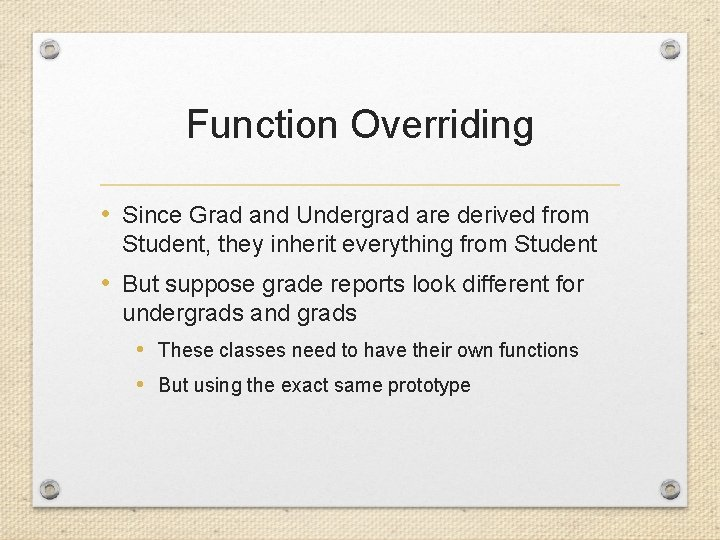Function Overriding • Since Grad and Undergrad are derived from Student, they inherit everything from Student • But suppose grade reports look different for undergrads and grads • These classes need to have their own functions • But using the exact same prototype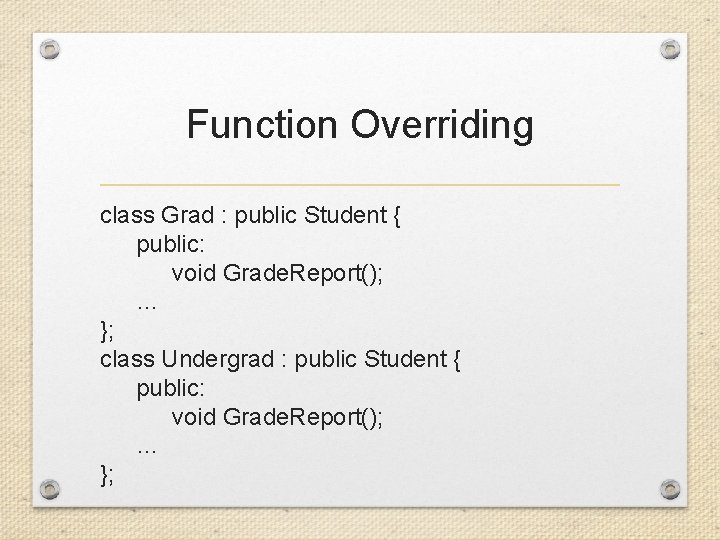Function Overriding class Grad : public Student { public: void Grade. Report(); … }; class Undergrad : public Student { public: void Grade. Report(); … };To Call the Parent Version void Student: : Grade. Report() { // processing done by parent function } void Grade: : Grade. Report() { // explicit call to parent function Student: : Grade. Report(); // other processing specific to Grad’s version }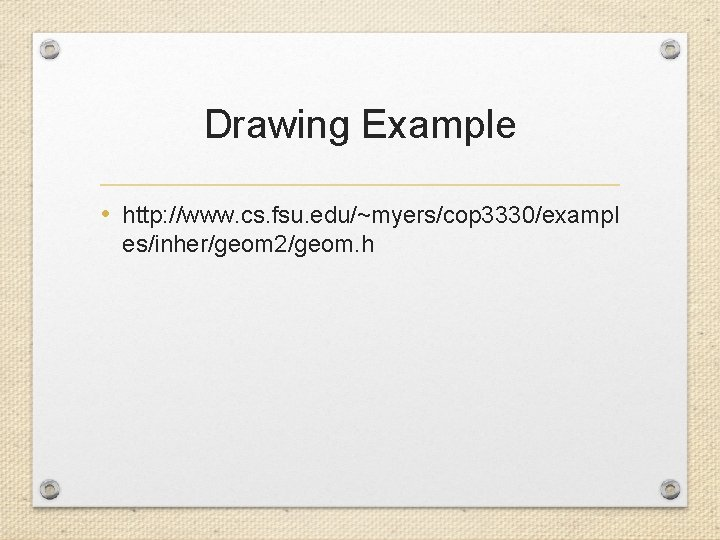Drawing Example • http: //www. cs. fsu. edu/~myers/cop 3330/exampl es/inher/geom 2/geom. h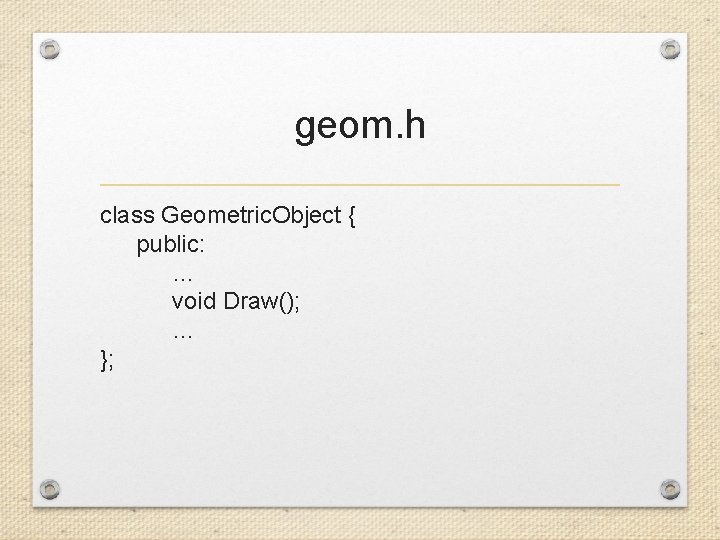geom. h class Geometric. Object { public: … void Draw(); … };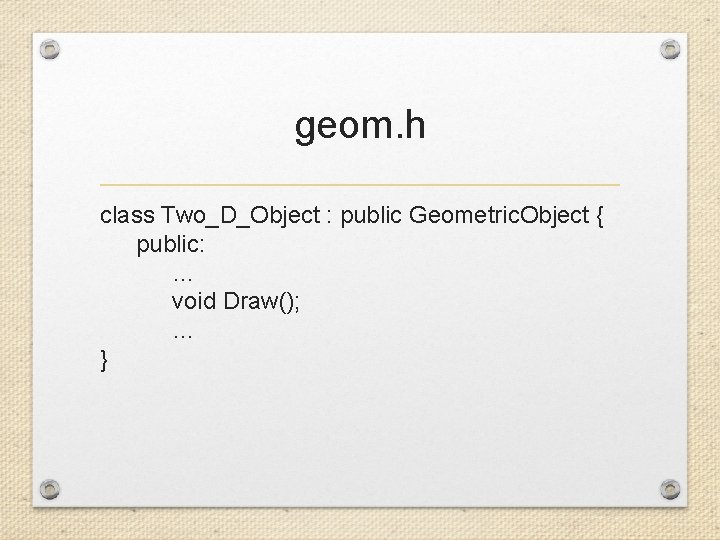geom. h class Two_D_Object : public Geometric. Object { public: … void Draw(); … }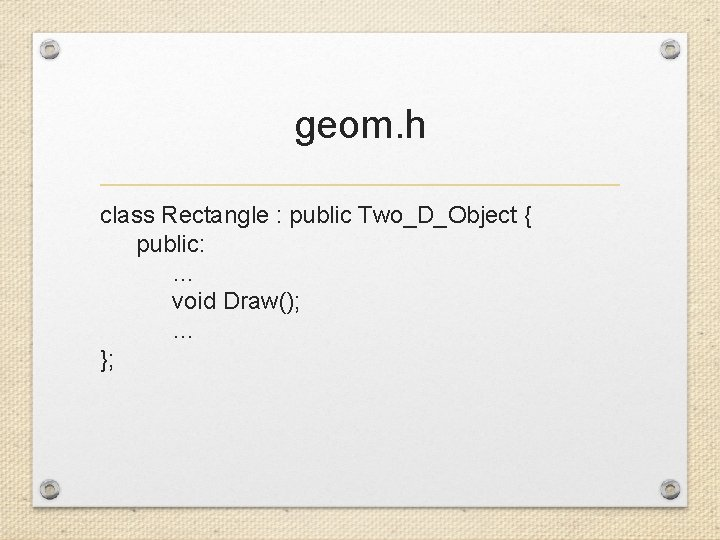geom. h class Rectangle : public Two_D_Object { public: … void Draw(); … };geom. h class Circle: public Two_D_Object { public: … void Draw(); … };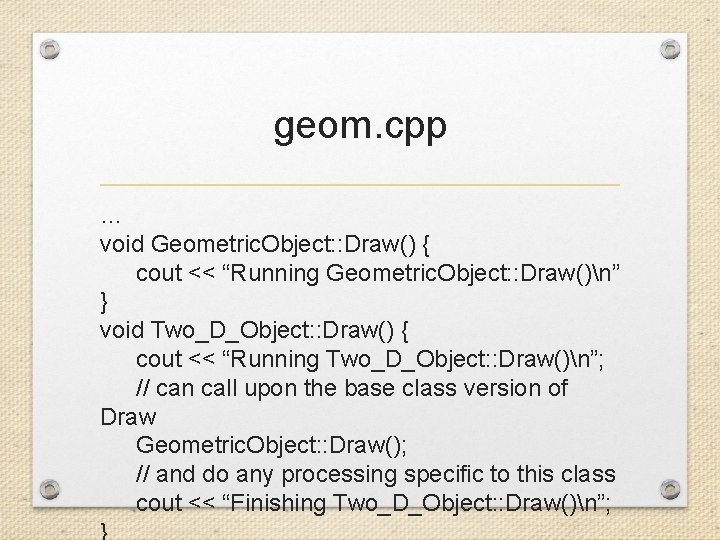geom. cpp … void Geometric. Object: : Draw() { cout << “Running Geometric. Object: : Draw()n” } void Two_D_Object: : Draw() { cout << “Running Two_D_Object: : Draw()n”; // can call upon the base class version of Draw Geometric. Object: : Draw(); // and do any processing specific to this class cout << “Finishing Two_D_Object: : Draw()n”; }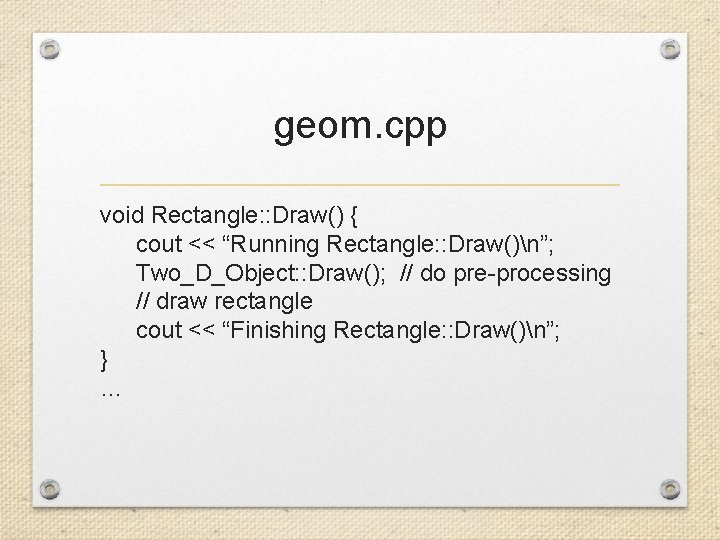geom. cpp void Rectangle: : Draw() { cout << “Running Rectangle: : Draw()n”; Two_D_Object: : Draw(); // do pre-processing // draw rectangle cout << “Finishing Rectangle: : Draw()n”; } …main. cpp #include <iostream> #include “geom. h” using namespace std; int main() { Rectangle r; … r. Draw(); return 0; }